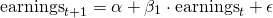# Earnings persistence

Earnings persistence refers to whether or not the earnings of a company are expected to recur or not. The higher the persistence of earnings, the more sustainable the earnings. Earnings that are comprised of a high proportion of non-recurring items are considered to be unsustainable.

On this page, we discuss how to classify items as non-recurring what if meant by classification shifting. We also discuss how to analyze the degree of persistence using a regression model. An Excel spreadsheet example is available for download at the bottom of the page.

## Earnings persistence definition

To analyze whether or not earnings are persistent, the first step is to classify items as non-recurring. This process is highly subjective. While shifting items between recurring and non-recurring will not impact total net income, it is a method used by management to make investors believe that the recurring part is higher than it actually is.

One commonly used method to overstate earnings is to mis-classify normal operating expenses as being expense from discontinued operations. Analysts should carefully analyze disclosures that reconcile pro-forma income with reported income. This way, investors can evaluate whether the items included as non-recurring are actually non-recurring. Another method to analyze whether or not earnings are of high quality, is to run a regression.

## Earnings persistence formula

The following earnings persistence formula can be used to gauge the persistence of a company’s earningsA higher value for the coefficient beta indicates that the company’s earnings are more persistent. Thus, the higher the beta, the better. The above formula is typically estimated using a simple ordinary least squares (OLS) regression.

## Earnings persistence example

Let’s apply the above formula using a numerical example. The following table illustrates how we can use Excel to estimate earnings persistence. The higher the beta, the better

## Summary

We discussed how to quantify the extent to which a company’s earnings are persistent. Another method to analyze persistence, is to look at accruals.